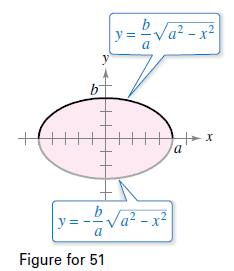Chapter 8.4, Problem 55E

Chapter
Section
Textbook Problem

# Area Find the Area enclosed by the ellipse x 2 a 2 + y 2 b 2 = 1 . shown in the figure.To determine
Area enclosed by the ellipse x2a2+y2b2=1.

Explanation

Given: The ellipse x2a2+y2b2=1:

Refer to the question for figure.

Solution: The area of the given ellipse is πab._

Explanation: x2a2+y2b2=1

y2=b2(1x2a2)y=baa2x2

Note that the ellipse is symmetrical about the x axis.

Therefore A=2aabaa2x2dx

Also note that ellipse is symmetrical about the y axis.

A=4aabaa2x2dx

i.e; Area is 4 times the area of the first quadrant of the ellipse.

Because a2x2 is a standard form

We use the trigonometric substitution:

x=asinθdx=acosθdθx=0sinθ=0θ=0x=asin

### Still sussing out bartleby?

Check out a sample textbook solution.

See a sample solution

#### The Solution to Your Study Problems

Bartleby provides explanations to thousands of textbook problems written by our experts, many with advanced degrees!

Get Started

#### In Exercises 516, evaluate the given quantity. log1616

Finite Mathematics and Applied Calculus (MindTap Course List)

#### In Exercises 69-74, rationalize the numerator. 70. x324

Applied Calculus for the Managerial, Life, and Social Sciences: A Brief Approach

#### Which property of real numbers is illustrated in each part of problem 3-6? 4. a) b)

Mathematical Applications for the Management, Life, and Social Sciences